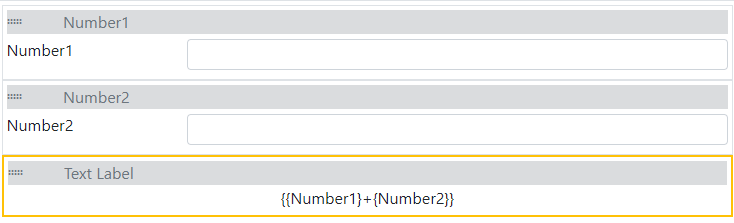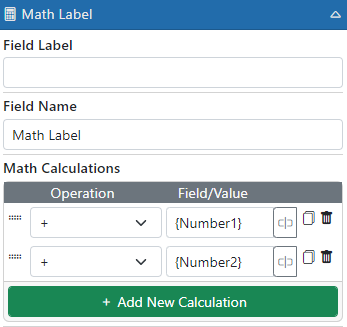(844) docmgt1 sales@docmgt.com

## E-form Calculations

Calculating values inside of E-forms is a common requirement. For instance, you may have a form for submitting expenses which needs to have all the expenses added together. It could further require its values be divided among different departments or categories. E-form calculations can help you display those values.

#### What Are E-form Calculations?

E-form calculations allow you to perform math within the form. We can add, subtract, multiply, divide, and more.  The syntax we use is basic JavaScript with placeholders for fields to get their values.

#### How to Perform E-form Calculations

Let’s say you need to add 2 fields – Number1 and Number2. You could place a label on the form and set its value to something like “Number1 + Number2” to get the value. However, you will need to enclose the field names inside of curly braces – i.e., {Number1} and {Number2} and wrap the equation in { and } as well. So, the final value for the label would be {{Number1}+{Number2}}. When the E-form is opened and values are being entered, {Number1} and {Number2} will be replaced with whatever is in those fields and then 1+2 will be computed. So, if you enter “5” into the Number1 field and “3” into Number2, then the label should show “8”.The above equation should work fine if you are using Number fields for Number1 and field2. However, there are some caveats. For instance, make sure to set the default value of 0 for the fields. Otherwise, if you leave one of them blank the equation might end up as “3+” which will not evaluate to anything. You also need to be careful if you are using regular text fields as users can enter in text that is not numeric. You might end up with “Five+3” which, once again, is not valid.

Another caveat is with currency. If the value has a dollar sign, then it needs to be removed for calculating. We have a built-in function to help with those – cleanNumericValue(). If you need to do the above calculation with text fields, we recommend doing it like this:

`{cleanNumericValue({Number1})+cleanNumericValue({Number2})}`

There are other built-in functions that you can use to help out in calculations:

#### String Functions

replaceAll(instr, find, replace) – Replaces all instances of find with replace in instr

stringContains(instr, containsStr) – Returns true if “instr” contains containsStr and false if not
This function is NOT case sensitive

#### Number Functions

getNumValue (instr) – If “instr” is a number then it is returned. If not, instr is sent into cleanNumericValue function to be cleaned to a number

cleanNumericValue (instr) – Removes all non-numeric characters from the incoming string

cleanDivision(val1, val2) – Divides val1 by val2 and if either value is null, 0 or non-numeric, it returns 0

tryParseFloat(value, default) – Tries to convert value to floating point number and if it cannot then it returns the default value

getFormattedNumber(value, places) – Formats the incoming value as a number with the specified number of decimal places

formatCurrency(value, places) – Formats the value using USD currency formatting

#### Date Functions

dateDiff(datefrom, dateto, interval) – Computes the number of intervals between datefrom and dateto.

Allowed “interval” values are:

• s, sec, second, seconds – Number of seconds
• n, min, minute, minutes – Number of minutes
• h, hour, hours – Number of hours
• d, day, days – Number of days
• m, mon, mons, month, months – Number of months
• y, yr, year, years – Number of years

timeDiff(timefrom, timeto, interval) – Computes the number of intervals between timefrom and timeto.

Allowed “interval” values are:

• n, min, minute, minutes – Number of minutes
• h, hour, hours – Number of hours
• Anything other value returns a string formatted as HH:MM

formatTime(hours, minutes, seconds) – Returns a date formatted using h:m:s format

See your online documentation for help on how to use these. You will find it in the Administration / E-forms section.

#### Math Label

One very helpful field type for E-form calculations is the Math Label. This label is built to do simple math for you and will automatically clean up number fields, currency, etc. You can even use 2 or more together to produce more complicated math.#### Saving Computed Values

If you need to save the results of your math when the E-form saves, you need to put the results to a field that can be saved such as Text or Hidden fields. The easiest way to save a calculated field is to place a Hidden field into your form and then use the “Get Value From” setting in the “Data Settings” panel to get it from the label. That way the calculated value is always in the hidden field to be saved. Of course, you can always place the calculation directly into a Hidden field if the users do not need to see it. If you decide to place the calculation it into a text field, make sure to disable the field so the users do not try to manually update the value.

## Final IMPORTANT Note

In the now-current release, you can get by with not using the outer { and } but in future releases that will no longer work. So, instead of this:

`cleanNumericValue({Number1})+cleanNumericValue({Number2})`

You need to use this:

`{cleanNumericValue({Number1})+cleanNumericValue({Number2})}`

Notice the outer { and } characters. This is because in future releases, we will ONLY be calculating inside the { and } signs and not the entire string.

### Related Articles

E-form Automation Events

Designing E-Forms

Public Access Portals